Next: Context sensitive spelling correction Up: Spelling correction Previous: Edit distance   Contents   Index

## k-gram indexes for spelling correction

To further limit the set of vocabulary terms for which we compute edit distances to the query term, we now show how to invoke the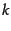-gram index of Section 3.2.2 (page) to assist with retrieving vocabulary terms with low edit distance to the query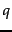. Once we retrieve such terms, we can then find the ones of least edit distance from.

In fact, we will use the-gram index to retrieve vocabulary terms that have many-grams in common with the query. We will argue that for reasonable definitions of many-grams in common,'' the retrieval process is essentially that of a single scan through the postings for the-grams in the query string.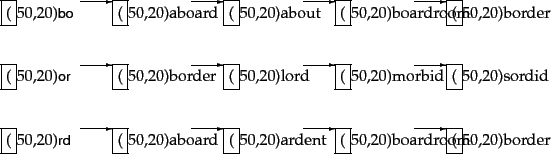The 2-gram (or bigram) index in Figure 3.7 shows (a portion of) the postings for the three bigrams in the query bord. Suppose we wanted to retrieve vocabulary terms that contained at least two of these three bigrams. A single scan of the postings (much as in Chapter 1 ) would let us enumerate all such terms; in the example of Figure 3.7 we would enumerate aboard, boardroom and border.

This straightforward application of the linear scan intersection of postings immediately reveals the shortcoming of simply requiring matched vocabulary terms to contain a fixed number of-grams from the query: terms like boardroom, an implausible correction'' of bord, get enumerated. Consequently, we require more nuanced measures of the overlap in-grams between a vocabulary term and. The linear scan intersection can be adapted when the measure of overlap is the Jaccard coefficient for measuring the overlap between two sets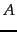and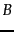, defined to be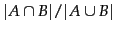. The two sets we consider are the set of-grams in the query, and the set of-grams in a vocabulary term. As the scan proceeds, we proceed from one vocabulary term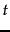to the next, computing on the fly the Jaccard coefficient betweenand. If the coefficient exceeds a preset threshold, we addto the output; if not, we move on to the next term in the postings. To compute the Jaccard coefficient, we need the set of-grams inand.

Since we are scanning the postings for all-grams in, we immediately have these-grams on hand. What about the-grams of? In principle, we could enumerate these on the fly from; in practice this is not only slow but potentially infeasible since, in all likelihood, the postings entries themselves do not contain the complete stringbut rather some encoding of. The crucial observation is that to compute the Jaccard coefficient, we only need the length of the string. To see this, recall the example of Figure 3.7 and consider the point when the postings scan for query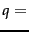bord reaches term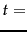boardroom. We know that two bigrams match. If the postings stored the (pre-computed) number of bigrams in boardroom (namely, 8), we have all the information we require to compute the Jaccard coefficient to be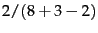; the numerator is obtained from the number of postings hits (2, from bo and rd) while the denominator is the sum of the number of bigrams in bord and boardroom, less the number of postings hits.

We could replace the Jaccard coefficient by other measures that allow efficient on the fly computation during postings scans. How do we use these for spelling correction? One method that has some empirical support is to first use the-gram index to enumerate a set of candidate vocabulary terms that are potential corrections of. We then compute the edit distance fromto each term in this set, selecting terms from the set with small edit distance to.Next: Context sensitive spelling correction Up: Spelling correction Previous: Edit distance   Contents   Index